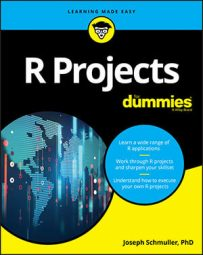##### R Projects For DummiesMachine Learning (ML) is a popular area. R provides a number of ML-related packages and functions. Here are some of them:

Machine Learning Packages and Functions

 Package Function What it does `rattle` `rattle()` Opens the Rattle Graphic User Interface `rpart` `rpart()` Creates a decision tree `rpart.plot` `prp()` Draws a decision tree `randomForest` `randomForest()` Creates a random forest of decision trees `rattle` `printRandomForests()` Prints the rules of a forest’s individual decision trees `e1071` `svm()` Trains a support vector machine `e1071` `predict()` Creates a vector of predicted classifications based on a support vector machine `kernlab` `ksvm()` Trains a support vector machine `base R` `kmeans()` Creates a k-means clustering analysis `nnet` `nnet()` Creates a neural network with one hidden layer `NeuralNetTools` `plotnet()` Draws a neural network `nnet` `predict()` Creates a vector of predictions based on a neural network